The PROC ADAPTIVEREG statement invokes the procedure.

Table 25.1 summarizes the options available in the PROC ADAPTIVEREG statement.

Table 25.1: PROC ADAPTIVEREG Statement Options

Option

Description

Data Set Options

Specifies the input SAS data set

Names a data set that contains test data

Names a data set that contains validation data

Computational Options

Sets optimization parameters for fitting generalized linear models

Sets the fuzzy comparison criterion in selection

Sets the singularity tolerance

Display Options

Sets the length of effect names in tables and output data sets

Controls plots produced through ODS Graphics

Displays detailed modeling information

Other Options

Requests the computation in single-threaded mode

Requests a data set that contains the design matrix

Sets the seed used for pseudo-random number generation

Specifies the number of threads for the computation

You can specify the following options.

DATA=SAS-data-set

specifies the SAS data set to be read by PROC ADAPTIVEREG. If you do not specify the DATA= option, PROC ADAPTIVEREG uses the most recently created SAS data set.

DETAILS<=(detail-options)>

requests detailed model fitting information. You can specify the following detail-options:

BASES

displays the "Bases Information" table.

BWDSUMMARY

displays the "Backward Selection Summary" table.

FWDSUMMARY

displays the "Forward Selection Summary" table.

FWDPARAMS

displays the "Forward Selection Parameter Estimates" table.

If you do not specify a detail-option, PROC ADAPTIVEREG produces all the preceding tables by default.

NAMELEN=number

specifies the length to which long effect names are shortened. The default and minimum value is 20.

NLOPTIONS(options)

specifies options for the nonlinear optimization methods if you are applying the multivariate adaptive regression splines algorithm to generalized linear models. You can specify the following options:

ABSCONV=r
ABSTOL=r

specifies an absolute function convergence criterion by which minimization stops when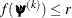, where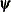is the vector of parameters in the optimization and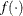is the objective function. The default value of r is the negative square root of the largest double-precision value, which serves only as a protection against overflows.

ABSFCONV=r
ABSFTOL=r

specifies an absolute function difference convergence criterion. For all techniques except NMSIMP, termination requires a small change of the function value in successive iterations,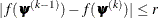wheredenotes the vector of parameters that participate in the optimization andis the objective function. The same formula is used for the NMSIMP technique, but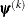is defined as the vertex with the lowest function value, and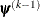is defined as the vertex with the highest function value in the simplex. The default value is r=0.

ABSGCONV=r
ABSGTOL=r

specifies an absolute gradient convergence criterion. Termination requires the maximum absolute gradient element to be small,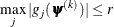wheredenotes the vector of parameters that participate in the optimization and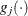is the gradient of the objective function with respect to the jth parameter. This criterion is not used by the NMSIMP technique. The default value is r = 1E–5.

FCONV=r
FTOL=r

specifies a relative function convergence criterion. For all techniques except NMSIMP, termination requires a small relative change of the function value in successive iterations,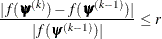wheredenotes the vector of parameters that participate in the optimization andis the objective function. The same formula is used for the NMSIMP technique, butis defined as the vertex with the lowest function value, andis defined as the vertex with the highest function value in the simplex. The default is r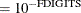, where FDIGITS is by default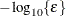andis the machine precision.

GCONV=r
GTOL=r

specifies a relative gradient convergence criterion. For all techniques except CONGRA and NMSIMP, termination requires the normalized predicted function reduction to be small,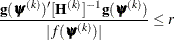wheredenotes the vector of parameters that participate in the optimization,is the objective function, and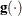is the gradient. For the CONGRA technique (where a reliable Hessian estimateis not available), the following criterion is used: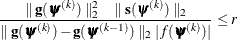This criterion is not used by the NMSIMP technique. The default value is r = 1E–8.

HESSIAN=hessian-options

specifies the Hessian matrix type used in the optimization of likelihood functions, if the Newton-Raphson technique is used. You can specify the following hessian-options:

EXPECTED

requests that the Hessian matrix in optimization be computed as the negative of the expected information matrix.

OBSERVED

requests that the Hessian matrix in optimization be computed as the negative of the observed information matrix. For many specified distribution families and link functions, the observed information matrix is equal to the expected information matrix.

The default is HESSIAN=EXPECTED.

MAXFUNC=n
MAXFU=n

specifies the maximum number of function calls in the optimization process. The default values are as follows, depending on the optimization technique:

• TRUREG, NRRIDG, and NEWRAP: 125

• QUANEW and DBLDOG: 500

• CONGRA: 1000

• NMSIMP: 3000

The optimization can terminate only after completing a full iteration. Therefore, the number of function calls that are actually performed can exceed the number that is specified by this option. You can select the optimization technique by specifying the TECHNIQUE= option.

MAXITER=n
MAXIT=n

specifies the maximum number of iterations in the optimization process. The default values are as follows, depending on the optimization technique:

• TRUREG, NRRIDG, and NEWRAP: 50

• QUANEW and DBLDOG: 200

• CONGRA: 400

• NMSIMP: 1000

These default values also apply when n is specified as a missing value. You can select the optimization technique by specifying the TECHNIQUE= option.

MAXTIME=r

specifies an upper limit of r seconds of CPU time for the optimization process. The time is checked only at the end of each iteration. Therefore, the actual run time might be longer than the specified time. By default, CPU time is not limited.

MINITER=n
MINIT=n

specifies the minimum number of iterations. The default value is 0. If you request more iterations than are actually needed for convergence to a stationary point, the optimization algorithms can behave strangely. For example, the effect of rounding errors can prevent the algorithm from continuing for the required number of iterations.

TECHNIQUE=keyword

specifies the optimization technique to obtain maximum likelihood estimates for nonnormal distributions. You can choose from the following techniques by specifying the appropriate keyword:

CONGRA

DBLDOG

performs a version of double-dogleg optimization.

NEWRAP

performs a Newton-Raphson optimization that combines a line-search algorithm with ridging.

NMSIMP

NONE

performs no optimization.

NRRIDG

performs a Newton-Raphson optimization with ridging.

QUANEW

performs a dual quasi-Newton optimization.

TRUREG

performs a trust-region optimization.

The default is TECHNIQUE=NEWRAP.

For more information about these optimization methods, see the section Choosing an Optimization Algorithm in Chapter 19: Shared Concepts and Topics.

forces single-threaded execution of the analytic computations. This overrides the SAS system option THREADS | NOTHREADS. Specifying this option is equivalent to specifying the NTHREADS=1 option.

OUTDESIGN<(options)>=SAS-data-set

creates a data set that contains the design matrix of constructed basis functions. The design matrix column names consist of a prefix followed by an index. The default naming prefix is _X. The default output is the design matrix of basis functions after backward selection.

You can specify the following options in parentheses to control the content of the OUTDESIGN= data set:

BACKWARDMODEL |BACKWARD

produces the design matrix for the selected model after the backward selection.

FORWARDMODEL |FORWARD

produces the design matrix for the selected model after the forward selection.

PREFIX=prefix

requests that the design matrix column names consist of a prefix followed by an index.

STARTMODEL

produces the design matrix for the initial model specified in the MODEL statement.

PLOTS <(global-plot-options)> <= plot-request <(options)>>
PLOTS <(global-plot-options)> <= (plot-request <(options)> <... plot-request <(options)>>)>

controls the plots produced through ODS Graphics. When you specify only one plot-request, you can omit the parentheses around the plot-request. For example:

plots=all
plots=components(unpack)
plots(unpack)=(components diagnostics)


ODS Graphics must be enabled before plots can be requested. For example:

ods graphics on;

model y=x1 x2;
run;

ods graphics off;


For more information about enabling and disabling ODS Graphics, see the section Enabling and Disabling ODS Graphics in Chapter 21: Statistical Graphics Using ODS.

You can specify the following global-plot-option, which applies to all plots that the ADAPTIVEREG procedure generates:

UNPACK |UNPACKPANEL

suppresses paneling. By default, multiple plots can appear in some output panels. Specify UNPACK to get each plot individually. You can also specify UNPACK as a suboption with COMPONENTS and DIAGNOSTICS.

You can specify the following plot-requests and their options:

ALL

requests that all default plots be produced.

COMPONENTS <(component-options)>

plots a panel of functional components of the fitted model. You can specify the following component-options:

COMMONAXES

specifies that the functional component plots use a common vertical axis except for contour plots. This enables you to visually judge relative effect size.

UNPACK |UNPACKPANEL

displays the component plots individually.

DIAGNOSTICS <(UNPACK |UNPACKPANEL)>

produces a summary panel of fit diagnostics that consists of the following:

• residuals versus the predicted values

• a histogram of the residuals

• a normal quantile plot of the residuals

• a residual-fit (RF) plot that consists of side-by-side quantile plots of the centered fit and the residuals

• response values versus the predicted values

You can request the five plots in this panel as individual plots by specifying the UNPACK suboption. The fit diagnostics panel is not produced for dependent variable with nonnormal distributions.

FIT <(NODATA |NOOBS)>

produces a plot of the predicted values against the variables that form the selected model. By default, a scatter plot of the input data is overlaid. You can suppress the scatter plot by specifying the NODATA | NOOBS option.

The plot is not produced if the number of variables in the selected model exceeds two. The plot is not produced for dependent variables with nonnormal distributions.

NONE

suppresses all plots.

SELECTION<(selection-panel-options)>

plots a panel of model fit criteria. The panel consists of two plots. The upper plot shows the progression of the model lack-of-fit criterion as the selection process proceeds. The lower plot shows the progression of the model validation criterion as the selection process proceeds. By default, the selection panel shows the progression for the backward selection process. You can specify the following selection-panel-options:

BACKWARDMODEL |BACKWARD

displays the progression of model fit criteria for the backward selection process.

FORWARDMODEL |FORWARD

displays the progression of model fit criteria for the forward selection process.

SEED=number

specifies an integer used to start the pseudorandom number generator for random cross validation and random partitioning of data for training, testing, and validation. If you do not specify a seed, or if you specify a value less than or equal to 0, the seed is generated from the time of day, which is read from the computer’s clock.

SELFUZZ=number
SELECTFUZZ=number

sets the fuzzy comparison criterion when PROC ADAPTIVEREG examines candidate basis functions in forward and backward selection stages. The fuzzy comparison criterion is also used in stepwise selection for CLASS variables. A candidate is considered to be the best one only when its improvement is better than the current optimum with the extra amount number. By default, number is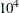times the machine epsilon. The default number is approximately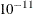on most machines.

SINGULAR=number
EPSILON=number

sets the tolerance for testing singularity of the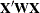matrix that is formed from the design matrix. Roughly, the test requires that a pivot be at least this number times the original diagonal value. By default, number is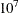times the machine epsilon. The default number is approximately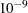on most machines.

TESTDATA=SAS-data-set

names a SAS data set that contains test data. This data set must contain all the variables specified in the MODEL statement. Furthermore, when a BY statement is used and the TESTDATA=data set contains any of the BY variables, then the TESTDATA= data set must also contain all the BY variables sorted in the order of the BY variables. In this case, only the test data for a specific BY group are used with the corresponding BY group in the analysis data. If the TESTDATA= data set contains none of the BY variables, then the entire TESTDATA = data set is used with each BY group of the analysis data.

If you specify a TESTDATA= data set, then you cannot also specify a PARTITION statement to reserve observations for testing.